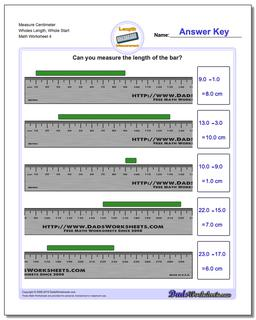# Math Worksheets: Metric Measurement: Metric Measurement: Measure Centimeter Wholes Length, Whole Start (Fourth Worksheet)## Measure Centimeter Wholes Length, Whole Start (Fourth Worksheet)

PropertyValue
DescriptionMeasure Centimeter Wholes Length, Whole Start: Measurement worksheets for measuring the length of an object placed at arbitrary whole centimeter positions on a metric ruler. These problems provide great applied practice for subtracting measurements. (Fourth Worksheet)
Resource TypeWorksheet